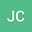•This work is focused on establishing sufficient conditions to guarantee the well-posedness of the following nonlinear fractional semidiscrete model \begin{equation*} \begin{cases} \mathbb D^\beta_t u(n,t)= B u(n,t) + f(n-ct,u(n,t)),\, &n\in\mathbb{Z}, \;t>0,\\ u(n,0)=\varphi(n),\; &n\in\mathbb{Z},\\ \end{cases} \end{equation*} under the assumptions that $\beta \in (0,1]$, $c>0$ some constant, $B$ is a discrete convolution operator with kernel $b\in\ell^1(\Z)$, which is the infinitesimal generator of the Markovian $C_0$-semigroup and suitable nonlinearity $f$. We present results concerning the existence and uniqueness of solution, as well as establishing a comparison principle of solutions according to respective initial values.